# Multiple choice questions on Kinetic theory of gases for Jee Main and Advanced

## Multiple choice questions with one or more answer

Question 1. Let A & B are two sample of ideal gases of equal mole .let T be the temperature of both the gas Let EA and EB are there total energy respectively .Let MA and MB are these respective molecular mass .which of these is true
a, EA >EB
b, EA < EB
c, EA =EB
d, none of these

Question 2. An Ideal gas undergoes an state change according to PV diagram. what is the value Vx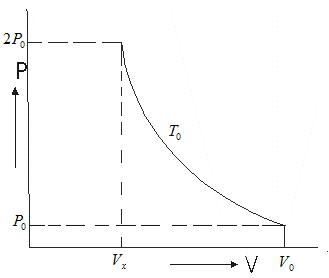a, V0/ 2
b, V0
c, 2V0
d, V0/ 4

A container contains N2 gas at T K. The no of moles of gas is n0. Consider it behaves like ideal gas. It rms speed is v0

Question 3.What is the total translational kinetic energy
a, (3/2 )n0RT
b, 3/2 RT
c, 1/2 RT
d, (1/2) n0RT

Question 4.Suppose the temperature of gas is tripled and N2 molecules dissociate into atom. Then what will be the rms speed of atom.
a, v0√6
b. √(6 v0)
c, v0√3
d, √(3 v0)

Question 5.if Vp denotes most probable velocity of N2 at T then which of these is correct
a, Vp : V0= √2 : √3
b, Vp : V0 = 1 : 1
c, Vp : V0 = 2 : 3
d, Vp : V0 = √2 : 3

## Multiple choice questions with one or more answer

Question 6 An ideal gas undergoes the process describe by equation
P = P0 - aV2
Where P0 , a are positive constant and V is the volume of one mole of gas
Maximum temperature attainable by gas
a, 2/3 (P0/R) √(P0/3a)
b, 3 P0 √P0/2Ra
c, (1/3R)√(P0/3a)
d, 4/3 (P0/R)√(P0/2)

Question 7. Let V, Vrms and Vp respectively denotes the mean speed, root mean square speed and most probable speed of the molecule in ideal monoatomic gas at absolute temperature T. The mass of the molecule
is m then
A, No molecule can have a speed greater the (2)1/2Vrms
B, No molecule can have a speed less the Vp / (2)1/2
C, Vp < V < Vrms
D, The average kinetic charge of a molecule (3/4 )mVp2

A flask contains Oxygen, Hydrogen & chlorine in the ration of 3:2:1 mixture at 27 °C
Molecular mass of Oxygen = 32
Molecular mass of Hydrogen = 2
Molecular mass of Chlorine = 70.9

Question 8.Find the ratio of average kinetic energy per molecule of Oxygen & Hydrogen
1, 1:2
2, 1:1
3, 1:16
4, 2:1

Question 9.find the ratio of average kinetic energy per molecule of Hydrogen & chlorine
1, 1:2
2, 1:1
3, 1:16
4, 1:1

Question 10.find the ratio of mean speed of Oxygen, chlorine, Hydrogen
a, 4:1 : (.45)1/2
b, 5:2 :(5)1/2
c, 1: (.45)1/2:4
d, 2 :(.45)1/2 : (1.5)1/2

## Multiple choice questions with one or more answer

Question 11.Suppose a container is evacuated to have just one molecule of a gas in it. Let Va & Vrms represent the average speed and rms speed of the molecule
a) Va > Vrms
b) Va < Vrms
c) Va = Vrms
d) Vrms is undefined

Question 12. The velocities of the molecules are v, 2v, 3v, 4v & 5v. The rms speed will be
a, 11v
b, v(11)1/2
c, v
d, 3.3v

Question 13.Equal Number of molecules of hydrogen & Oxygen are contained in a vessel at one atmosphere
pressure. The ratio of the collision frequency of hydrogen molecules to the of Oxygen molecules on the
container
a,1:4
b,4:1
c, 1:16
d, 16:1

Question 14.The prefect gases A, B & C having masses m1, m2& m3 at temperature T1, T2 & T3 are mixed without any loses of internal energy of the molecules. The molecules weight of the gases is M1, M2, M3 what
is the final temperature of mixture.
a, [(m1/M1)T1 + (m2/M2)T2 + (m3/M3)T3]/(m1/M1+m2/M2+m3/M3)
C, [(M1/m1)T1 + (M2/m2)T2 + (M3/m3)T3]/(M1/m1+M2/m2+M3/m3)
b, [(m1)T1 + (m2)T2 +(m3)T3]/(m1+m2+m3)
d, [(M1)T1 + (M2)T2 + (M3)T3]/(M1+M2+M3)

Question 15. An ideal gas under goes an process show in Pv diagram.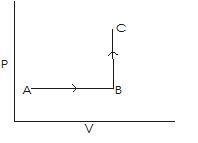Which of the diagram represent it on V-T diagram
a.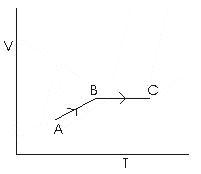b.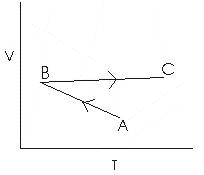c.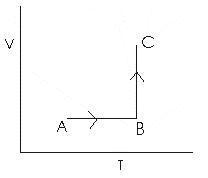d.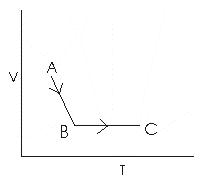Question 16 A gas mixture consist of molecules of type A, B, C, D with molecular
masses Ma > Mb > Mc >Md
Two statement are drawn from it
Statement A, Average kinetic energy of four type of gases in the mixture are in the ratio
Ea/1 =Eb/1 =Ec/1 =Ed/1
Statement B, Rms speed of molecules of the four types are in the order if V is the rms speed
VD > VC > VC > VA
which one of following is true
a, Only A correct
b, Only B correct
c, A & B both are correct
d, A & B both are wrong

Question 17. There are two statement
Statement A. Equal volumes of all gases at the same temperature T and pressure P contain an equal no of Molecules
Statement B,.the no of molecules in one mole of any gas is 6.0255 * 1022.
which one of the following is correct
a. A and B both
b. A only
c B only
d. A and B both are incorrect

Question 18.There are two statement about Ideal gases
Statement A The Vrms of gas molecules depends on the mass of the gas molecule and the temperature
Statement B The Vrms is same for all the gases at the same temperature
which one of the following is correct
a. A and B both
b. A only
c. B only
d. A and B both are incorrect